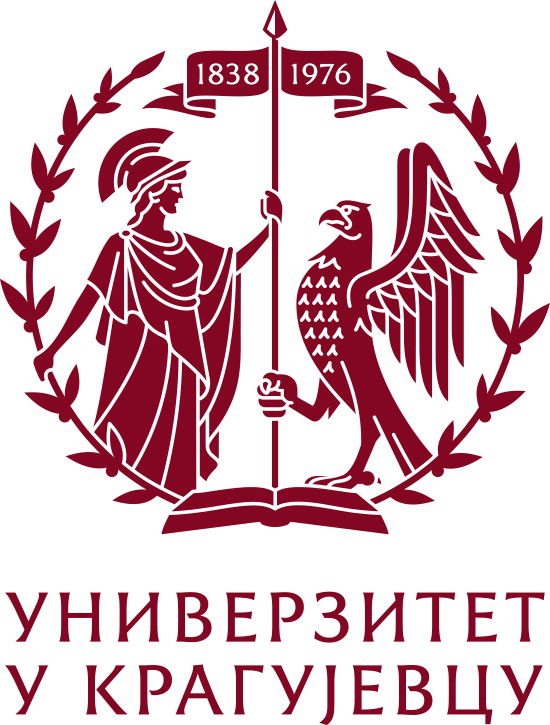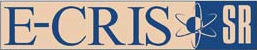Please use this identifier to cite or link to this item: `https://scidar.kg.ac.rs/handle/123456789/9267`
 Title: Laplacian energy of union and cartesian product and laplacian equienergetic graphs Authors: Ramane H.Gudodagi G.Gutman, IvanJournal: Kragujevac Journal of Mathematics Issue Date: 1-Jan-2015 Abstract: The Laplacian energy of a graph G with n vertices and m edges is defined as LE(G) = ∑ni=1 |μi-2m/n|, where μ1, μ2,...,μn are the Laplacian eigenvalues of G. If two graphs G1 and G2 have equal average vertex degrees, then LE(G1 ∪ G2) = LE(G1) + LE(G2). Otherwise, this identity is violated. We determine a term Ξ, such that LE(G1) + LE(G2) - Ξ ≤LE(G1 ∪ G2) ≤ LE(G1)+LE(G2)+Ξ holds for all graphs. Further, by calculating LE of the Cartesian product of some graphs, we construct new classes of Laplacian non-cospectral, Laplacian equienergetic graphs. URI: https://scidar.kg.ac.rs/handle/123456789/9267 Type: Article DOI: 10.5937/KgJMath1502193R ISSN: 14509628 SCOPUS: 84951059456 Appears in Collections: Faculty of Science, Kragujevac
[ Google Scholar ]

47